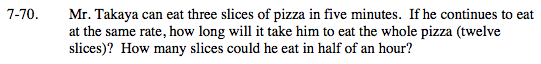### Home > MC2 > Chapter 7 > Lesson 7.2.1 > Problem7-70

7-70.$\text{If Mr. Takaya's rate is: }\frac{3\text{ slices}}{5\text{ minutes}}$

What can you multiply that by to get 12 slices?

$\frac{3\text{ slices}}{5 \text{ minutes}}\cdot \frac{4}{4}=\frac{12 \text{ slices}}{? \text{ minutes}}$

5 minutes (4) = 20 minutes
Mr. Takaya can eat the whole pizza in 20 minutes.

Try using this same method to find the number of slices Mr. Takaya can eat in half an hour (30 minutes).